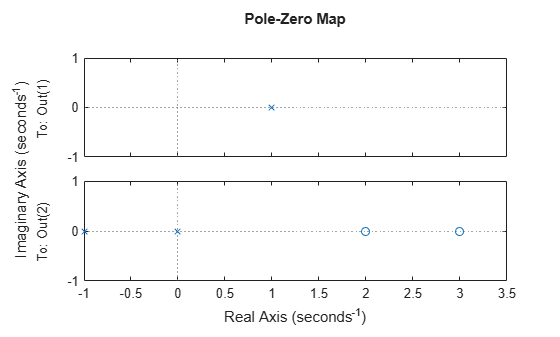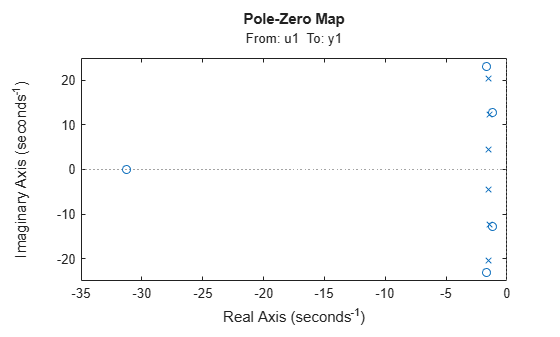# iopzmap

Plot pole-zero map for I/O pairs of model

## Syntax

```iopzmap(sys) iopzmap(sys1,sys2,...) ```

## Description

`iopzmap(sys)` computes and plots the poles and zeros of each input/output pair of the dynamic system model `sys`. The poles are plotted as x's and the zeros are plotted as o's.

`iopzmap(sys1,sys2,...)` shows the poles and zeros of multiple models `sys1`,`sys2`,... on a single plot. You can specify distinctive colors for each model, as in `iopzmap(sys1,'r',sys2,'y',sys3,'g')`.

The functions `sgrid` or `zgrid` can be used to plot lines of constant damping ratio and natural frequency in the s or z plane.

For model arrays, `iopzmap` plots the poles and zeros of each model in the array on the same diagram.

## Examples

collapse all

Create a one-input, two-output dynamic system.

`H = [tf(-5 ,[1 -1]); tf([1 -5 6],[1 1 0])];`

Plot a pole-zero map.

`iopzmap(H)``iopzmap` generates a separate map for each I/O pair in the system.

View the poles and zeros of an over-parameterized state-space model estimated from input-output data. (Requires System Identification Toolbox™).

```load iddata1 sys = ssest(z1,6,ssestOptions('focus','simulation')); iopzmap(sys)```The plot shows that there are two pole-zero pairs that almost overlap, which hints are their potential redundancy.

## Tips

For additional options for customizing the appearance of the pole-zero plot, use `iopzplot`.